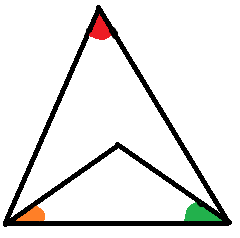# Vanish the camouflage

Geometry Level 3In the above figure , the red angle equals the sum of the orange angle and green angle.If $R_1$ is the circumradius of small triangle and $R_2$ is circumradius of bigger triangle , compare $R_1 , R_2$.

×